# How To Calculate Land Area Or Plot Area

Contents

## How To Calculate Land Area Or Plot Area:

Sometimes we may need to know the area of any land or plot for various reasons such as buying, selling of land, or constructing house/building etc. In this article, I will explain how to calculate land area or plot area for a site.

Area Formula For Different Shapes are given below:But for complex shaped land you cannot easily calculate the area. In that case, we use HERON’s Formula which is given below:

### Land Area Calculation: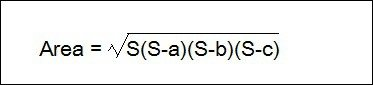Where S = Half of the Perimeter of the triangle

S = (a + b + c)/2

a = Distance of AB

b = Distance of BC

c =  Distance of AC

Let’s take an example,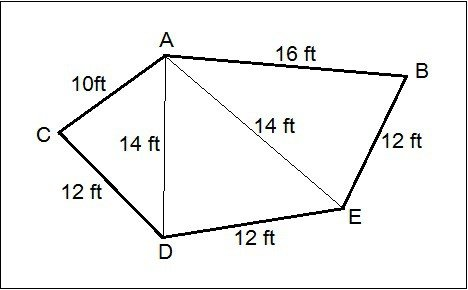First of all, divide the land area into minimum possible no. of triangle. Then measure all the required distance (like AB, AC, AE etc) by using a tape or chain.

1. For △ ACD:

AC = 10 feet.

CD = 12 feet.

S = (a + b + c)/2 = (10 + 12 + 14)/2 = 18 feet.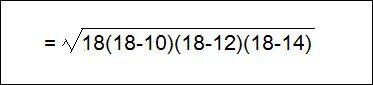##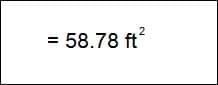2. For △AED:

AE = 14feet.

DE = 12 feet.

S = (a + b + c)/2 = (14+14+12)/2 = 20 feet.##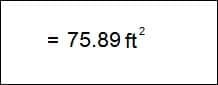3. For △AEB:

AB = 16 feet.

BE = 12 feet.

AE = 12 feet.

S = (a + b + c)/2 = (16 + 12 + 12)/2 = 20 feet.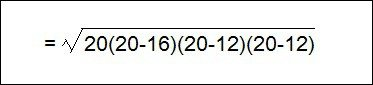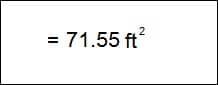Total Area Of Land = 58.78 + 75.89 + 71.55 = 206.22 ft²

Note:

This method does not give appropriate result for curve shaped area, for curve shaped area we need to use difinite integral method.

I hope you have now enough knowledge about plot area calculation. If you have any problem or question you can ask in the comments.

##### Center Line Method Of Estimation

How To Calculate Soil/Sand For Plot Filling

### 11 thoughts on “How To Calculate Land Area Or Plot Area”

1. I need more details about calculating land area

• Please correct the below point.

S = Semi-Perimeter of the Triangle.

Semi-Perimeter is half of its Perimeter.

2. Please explain it more to me ,I don’t understand it .it is principal scope of our field.

3. Thank for your Sharing about the Civil engineering Details of areas Measurement

4. Job ke liye apply kar raha hun electrician ka kam ho please bataiye p

5. my plot size is on street side 29.3 ft, opposite 22.3 ft., right side 32.6 ft and left side 15.6 please calculate area.

6. How to calculate 47/1140 ownership shares into kanals and marlas

7. How to calculate 47/1140 ownership shares into kanals and marlas
Thanks Ijaz
Plano, Texas. USA